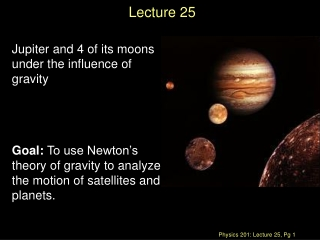DownloadDownload PresentationLecture 25

# Lecture 25

Download Presentation## Lecture 25

- - - - - - - - - - - - - - - - - - - - - - - - - - - E N D - - - - - - - - - - - - - - - - - - - - - - - - - - -
##### Presentation Transcript

1. Lecture 25 Jupiter and 4 of its moons under the influence of gravity Goal: To use Newton’s theory of gravity to analyze the motion of satellites and planets.

2. Exam results • Mean/Median 59/100 • Nominal exam curve A 81-100 AB 71-80 B 61-70 BC 51-60 C 31-50 D 25-30 F below 25

3. Newton’s Universal “Law” of Gravity Newton proposed that every object in the universe attracts every other object. The Law: Any pair of objects in the Universe attract each other with a force that is proportional to the products of their masses and inversely proportional to the square of their distance

4. Newton’s Universal “Law” of Gravity “Big” G is the Universal Gravitational Constant G = 6.673 x 10-11 Nm2 / kg2 Two 1 Kg particles, 1 meter away Fg= 6.67 x 10-11 N (About 39 orders of magnitude weaker than the electromagnetic force.) The force points along the line connecting the two objects.

5. Little g and Big G Suppose an object of mass m is on the surface of a planet of mass M and radius R. The local gravitational force may be written as where we have used a local constant acceleration: On Earth, near sea level, it can be shown that gsurface ≈ 9.8 m/s2.

6. g varies with altitude • With respect to the Earth’s surface

7. At what distance are the Earth’s and moon’s gravitational forces equal • Earth to moon distance = 3.8 x 108 m • Earth’s mass = 6.0 x 1024 kg • Moon’s mass = 7.4 x 1022 kg

8. The gravitational “field” • To quantify this invisible force (action at a distance) even when there is no second mass we introduce a construct called a gravitational “force” field. • The presence of a Gravitational Field is indicated by a field vector representation with both a magnitude and a direction. M

9. Compare and contrast • Old representation • New representation M

10. Gravitational Potential Energy Recall for 1D: When two isolated masses m1 and m2 interact over large distances, they have a gravitational potential energy of The “zero” of potential energy occurs at r = ∞, where the force goes to zero. Note that this equation gives the potential energy of masses m1 and m2 when their centers are separated by a distance r.

11. A plot of the gravitational potential energy • Ug= 0 at r = ∞ • We use infinity as reference point for Ug(∞)= 0 • This very different than • U(r) = mg(r-r0) • Referencing infinity has some clear advantages • All r allow for stable orbits but if the total mechanical energy, K+ U > 0, then the object will “escape”.

12. It is the same physics • Shifting the reference point to RE.

13. Gravitational Potential Energy with many masses • If there are multiple particles • Neglects the potential energy of the many mass elements that make up m1, m2 & m3 (aka the “self energy”

14. Dynamics of satellites in circular orbits Circular orbit of mass m and radius r • Force: FG= G Mm/r2 =mv2/r • Speed: v2 = G M/r (independent of m) • Kinetic energy: K = ½ mv2 = ½ GMm/r • Potential energy UG = - GMm/r UG = -2 K This is a general result • Total Mechanical Energy: • E = K + UG = ½ GMm/r -GMm/r E = - ½ GMm/r

15. Changing orbits • With man-made objects we need to change the orbit Examples: 1. Low Earth orbit to geosynchronous orbit 2. Achieve escape velocity • 1. We must increase the potential energy but one can decrease the kinetic energy consistent with

16. Changing orbits • Low Earth orbit to geosynchronous orbit • A 470 kg satellite, initially at a low Earth orbit of hi = 280 km is to be boosted to geosynchronous orbit hf = 35800 km • What is the minimum energy required to do so? (rE = 6400 km, M = 6 x 1024 kg

17. Escaping Earth orbit • Exercise: suppose an object of mass m is projected vertically upwards from the Earth’s surface at an initial speed v, how high can it go ? (Ignore Earth’s rotation)

18. Escaping Earth orbit • Exercise: suppose an object of mass m is projected vertically upwards from the Earth’s surface at an initial speed v, how high can it go ? (Ignore Earth’s rotation)

19. Escaping Earth orbit • Exercise: suppose an object of mass m is projected vertically upwards from the Earth’s surface at an initial speed v, how high can it go ? (Ignore Earth’s rotation) implies infinite height

20. Some interesting numbers • First Astronautical Speed • Low Earth orbit: v = 7.9 km/s • Second Astronautical Speed • Escaping the Earth: v = 11 km/s • Third Astronautical Speed • Escaping Solar system: v= 42 km/s

21. Next time • Chapter 14 sections 1 to 3, Fluids